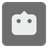Fortress Cannons
Simple+
English

You have found the enemy. It's the legions of chaos. You are the artillery commander of the fort and it’s up to you to give commands so that each cannon shoot at the legions.

The map used in this mission is a hex grid with a maximum size of 12x9, where each hex is numbered from A1 to L9. (A - L indicate a column, and 1 - 9 indicate a row.)

You are given three arguments: the first one is the position of your fort (a string), the second is the specification of each cannon(a list of tuples), and the third is the position of enemies (a set of strings).

• The details of the specification of each cannon (tuple) are:
• The first value is the firing-arc. This is the shooting area for the cannon (see the following images). The value is either 0 or 60, or 120.
• The second value is minimum-range. The cannon can shoot at this distance or more.
• The third value is maximum-range. The cannon can shoot at distance or less.
0 60 120* if cannon's direction is "N"

You should return a list of directions ('N', 'NE', 'SE', 'S', 'SW' or 'NW') for each cannon.

• The order is the same as the input value of the cannon's specification.
• All enemies must be in the shooting area and at the cannon’s range.
• If the above is impossible, return None.
• A cannon that doesn’t work may exist. (In this case, it may be in any direction.)

Example:

```fortress_cannons('F5', [(0, 1, 4)], {'F2'}) == ['N']
fortress_cannons('F5', [(120, 3, 4)], {'F3'}) is None
fortress_cannons('F5', [(0, 2, 6), (120, 1, 3), (60, 1, 4)],{'L2', 'D3', 'C6', 'E9'}) == ['NE','NW','S']
```Input: Three arguments. The first one is the position of your fort (as a string). The second is the specification of cannons (as a list of tuples; each tuple has 3 integers: firing-arc, minimum-range, and maximum-range). The third is the position of enemies (as a set of strings).

Output: Directions (as a list of strings).

How it is used:
For designing tactical wargames.

Precondition:

• 'A1' ≤ fort, enemy ≤ 'L9'
• fort not in enemies
• firing-arc in (0, 60, 120)
• 1 ≤ minimum-range ≤ maximum-range
• 1 ≤ len(cannons) ≤ 4